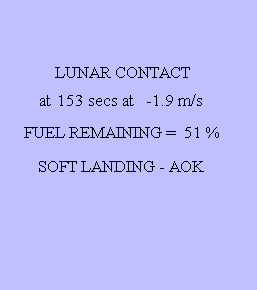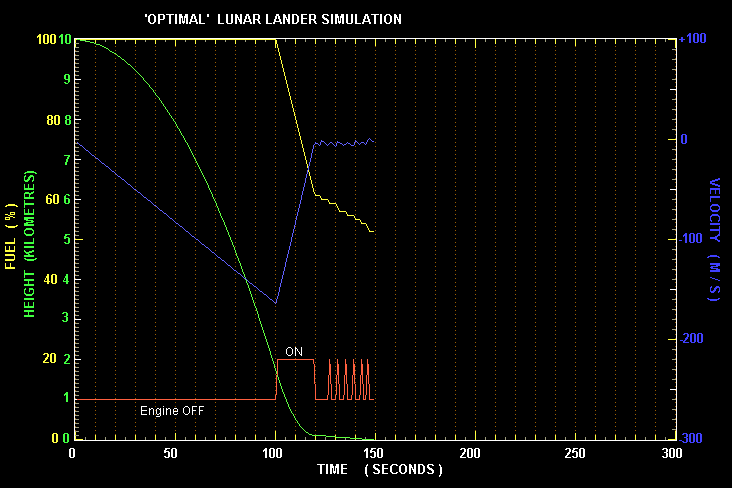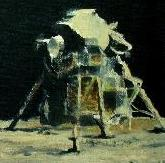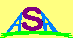### SIMULATING A LUNAR LANDING

INTRODUCTION

In this age of computer games there are many highly developed programs that simulate space flight in one form or another. Some of these are educational, but many are games with no relationship to the laws of planetary or interplanetary spaceflight.

One of the earliest 'space' games was known as 'lunar lander'. Without the graphics of todays computers, the modest requirements of this simulation could be implemented on the earliest PC's and even the early simple programmable calculators. The simulation is based on simple physics - the equations of motion under a constant acceleration, that are attributable to Galileo. These three equations are termed G1, G2 and G3. Only a few lines of code are needed to implement the core simulation. All the rest is window dressing - whether it be the simplest text interaction or a full graphical interface with sound effects.

Our presentation here is devoted to explaining the educational side of the simulation - the physics and mathematics involved. A basic program is presented and discussed as a core around which a more interesting simulation might be developed.

THE MODEL

The aim of the simulation is to start with a spacecraft (a lunar lander) at a height of h = H above the lunar surface - or the surface of any planet or moon without an atmosphere. If an atmosphere was present, the equations would become more complicated.

We assume that initially the craft is in free fall. This means that it is falling freely toward the lunar surface with an acceleration equal to the lunar gravitational acceleration. In this state the astronauts inside will be weightless. This is because although they are being acted upon by lunar gravity they are falling at an acceleration that that gravitational force produces.

At the initial height H we assume that the lander velocity is zero ( v = 0 ), although there is no reason why it could not be set to any other value (either positive - upward directed, or negative - downward directed).

The situation is illustrated in the figure below:There are only two conditions. The first is where the lander is in free fall and its acceleration a = -g where g is the value of the lunar gravitational acceleration. Because this is directed down toward the lunar surface and we have taken the positive direction of all our vectors to be up, the acceleration of the craft is negative or toward the surface of the moon. (Note that a vector is a physical quantity that has both a magnitude and a direction. In our case the direction can only be up (+) or down (-) ).

In this first condition, the spacecraft will accelerate toward the lunar surface, the velocity will increase in a negative direction and the height will decrease.

In the second condition the engine of the lander is firing. This produces a thrust which adds an upward acceleration we will call A. Although it is not important for our simulation, A = T / m where T is the thrust produced and m is the mass of the spacecraft. (this is Newton's second law). We are going to assume that this thrust is a constant. That is, the engine is either off or on. However, with a control input to a games port say, it would be quite easy to implement a variable thrust without any needed change in the equations.

The lander has limited fuel f = F and the rate of using the fuel is directly proportional to the time that the engine is on. The aim of the simulator of course, is to soft land the spacecraft on the lunar surface before the fuel runs out (ie f = 0 ).

To implement the simulation we consider the motion of the lander in small segments of time Δt. This will usually be the time it takes the computer to execute one pass through the iterative loop (ie to do the calculations below once and then print them out, before returning to do the same thing again). If we have access to the real-time clock in the computer, then our simulation will be a real-time simulation - that is, everything will happen exactly as it would in real life.

The equations that we must compute for each small amount of time are:

v = vo + a Δt       . . (Equation 1 . . . G1)

h = ho + vo Δt + a (Δt)2 / 2       . . (Equation 2 . . . G2)

If the engine is off we substitue -g for a, and if the engine is on we subtitute A - g for a, as long as the fuel is not zero. When the engine is on we must subtract a quantity Δf Δt from the remaining fuel f. We must also compute the new time. So altogether we have two more equations:
f = fo - Δf Δt     (only if the engine is on)       . . (Equation 3)

t = to + Δt       . . (Equation 4)

At the end of these calculations we print out the current time, height, velocity and fuel, until the lander hits the lunar surface. If it hits the surface with a velocity v > vs we declare a safe/soft landing. If not we have a crash. Notice that to hit the surface the velocity must be negative (you cannot land going up). So vs will have to be a small negative value. We might allow say vs = - 5 metres per second to be the maximum (negative) impact velocity for a safe landing.

We also have to choose the acceleration that the engine produces. A powerful engine might have an acceleration of 10 m/s2. This would be about six times the gravitational acceleration of the moon (and equal to the gravitational acceleration of the Earth). A less powerful engine might provide only twice the lunar acceleration ( ie 3.2 m/s2 ). The engine acceleration is of course always directed upward (+), but the total acceleration of the ship when the engine is on is A - g. It is interesting to note however, that the astronauts experience the full acceleration A, be it 10 or 3.2 m/s2 At A = 10, the astronauts will feel their full weight as if back on Earth. After a prolonged period of weightlessness in travelling to the moon, this much sudden acceleration will not be pleasant. One US Gemini astronaut likened it to being kicked hard in the stomach.

IMPLEMENTATION

A simple implementation of the above model is given in the QBASIC source code LUNSIM.BAS . Output displays both during the simulation and at the end are shown below:PLOTTING THE SIMULATION

To be more educational you really need to have a record of how you have flown the lander in past simulations, so that you can see what works and what doesn't. This can be done easily by storing the flight variables (height, velocity, acceleration and fuel) in an array second by second. At the end of the simulation the array can be written to a file where it can then be plotted at leisure.

This can be implemented by first declaring an array lundat(300,4). Then at the end of the simulation loop add the statements of the form:

``` time_index = int ( time )
lundat(time_index,1) = height
lundat(time_index,2) = velocity
lundat(time_index,3) = acceleration
lundat(time_index,4) = fuel
```
At the end of the simulation a comma separated variable (CSV) file can be created using code similar to:
``` open "lundat.csv" for output as #1
for tm = 1 to endtime
print #1, using "###.### , ####.# , ###.# , ###.#"; lundat(tm,1); lundat(tm,2); lundat(tm,3); lundat(tm,4)
next tm
```
This file can be plotted using XCEL or another plotting program. A plot of a flight is shown below.GRAPHICAL SIMULATION

To improve the simulation one can add 'meters' showing the parameters of height, velocity and fuel in analog format. Especially in situations where parameters are changing rapidly the eye-brain combination responds better to an analog presentation than a digital one. The simplest type of analog indicator is a bar graph. More sophisticated ones would be speedometer type gauges.

The other way to improve would be to add sounds. The current simulation has a sound for the engine, but warning sounds indicating ground proximity or high velocity might be added. Both meters and sounds are moderately easy to implement. Another simple change would be to incorporate a joystick and allow a variable throttle to the engine.

More sophisticated additions would be to have a central screen displaying an image of the lunar surface, and have this move in response to a 2D joystick which would impart a velocity in the north-south and east-west directions. This type of simulation requires a lot more skill, as not only do you have to land softly, but you must also land in a certain area. The simulation itself also requires a lot more programming skill.

THE OPTIMUM LANDING STRATEGY

It is probably a good idea to play with the simulation and get a feel for it before you read any further.

There is of course a minimum time and a minimum amount of fuel that you need to land softly on the lunar surface. This optimal landing strategy can be computed by using the last of the three Galilean equations of motion under a constant acceleration. This equation states that:

Δv2 = 2 a Δd       . . [ G3 ]
In this equation the time parameter has been eliminated (by substitution of t from G1 into G2), and we are left with a relation that connects velocity with distance.

We know consider the landing problem in two phases. The first phase involves allowing the spacecraft to descend in free fall toward the surface. Let the distance that it falls in this phase be df. During this time, from the above equation, it acquires a velocity:

vf2 = 2 g df
We now fire the rocket engine to reduce this velocity back to zero for a soft landing. This phase of the flight will occur over a distance de. We have to reduce the velocity we acquired during free fall vf back to zero. So we can again apply G3 to give:
vf2 = 2 ( A - g ) de
Putting these two expressions together we have:
g df = ( A - g ) de
But we know that to make a soft landing these two distances must add to the total height we started out from ( ie H ). So:
de + df = H

If we let the ratio of the accelerations be:

α = ( A - g ) / g
then if we put the two conditions together, we find that the optimum free fall distance is:
df = α H / ( 1 + α ) = ( A - g ) H / A
and
de = H - df
If we use the values g = 1.6 m s-2 and H = 10 km, we find that de = 1.6 km (or 1600 metres).

Thus for an optimum flight we allow the spacecraft to descend a distance of 8.4 km until it is 1.6 km above the lunar surface, at which time we fire the engines until we hit the lunar surface with zero velocity. A graph showing this flight profile is shown below.In practice, the lander velocity is highly negative (~ 166 m/s) at 1.6 km altitude and the height is rapidly decreasing. It it thus necessary to fire almost as soon as you pass the 1.7 km mark or it will be too late. As you near the surface you must shut down the engine and then quickly pulse it several times to keep your velocity above -5 m/s (but never above 0 m/s) during the last few metres of descent.

FURTHER EXPLORATIONS

There are several simple modifications that can be made to the basic lunar lander presented here to explore other aspects of spaceflight.

The gravitational acceleration can be changed to say that of Mars ( 3.7 m s-2 ) to examine how much extra fuel is needed for a soft landing - compared to that for the Moon. Comparison of optimum times is interesting also. Maybe you can work out a general formula that allows you to compute the fuel and landing time for any value of g?

Another exploration involves leaving the moon. How much fuel is needed to leave the Moon's gravitational influence. There is a quantity known as the escape velocity of a body, but this is the instantaneous velocity that would be needed to be given an object on the surface to fly to infinity. However, in practice, this situation can never be achieved, and even if it could, would result in death for astronauts - people don't like the very high accelerations necessary to achieve this.

In actual practice, when the rocket engine is fired for lift-off, the spacecraft velocity is slowly built up. The simulation can show what happens. Escaping from a planet means essentially that you reach a long distance away from the planet with a velocity just barely greater than zero. What do we mean by a large distance. Well, in the case of the Moon we are helped by the Earth. Determine the neutral gravitation point between the Earth and the Moon, and plan to reach this point with a small positive velocity.

If you want to be really realistic you should reduce the value of g as h increases - it will vary from 1.6 to 0 in an inverse square law fashion as you go from the surface to the neutral point. Be prepared however, in a real-time simulation such as this to take quite some time to reach that point!

In real-life, once you get to this distance, the Earth's gravity will take over and slowly draw you toward it and at the right time you can maneuver into Earth orbit.Australian Space Academy